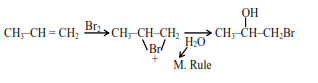# Given below are two statements: one is labelled as`
Question:

Given below are two statements : one is labelled as Assertion (A) and the other is labelled as Reason (R). Assertion (A) : Treatment of bromine water with propene yields 1-bromopropan-2-ol.

Reason (R) : Attack of water on bromonium ion follows Markovnikov rule and results in 1 -bromopropan-2-ol.

In the light of the above statements, choose the most appropriate answer from the options given below:

1. Both (A) and (R) are true but (R) is NOT the correct explanation of (A)

2. (A) is false but (R) is true.

3. Both (A) and (R) are true and (R) is the correct explanation of (A)

4. (A) is true but (R) is false

Correct Option: , 3

Solution:Its IUPAC name 1-bromopropan-2-ol

A and $R$ are true and $(R)$ is the correct explanation of (A)